# MacMullin Number Determination

A battery consists mainly of a positive and a negative electrode and electrolyte. Ionic and electronic conductivity occur at the two electrodes but only the former in the electrolyte.

The most widely used electrolytes are liquid, and therefore need solid supporting material to separate the electrodes physically to avoid short circuiting. Most commonly, the separator is a sheet of polymer, with a porous structure, which is soaked in electrolyte before the battery is assembled. The sheet physically separates the electrodes while the pores bring both into contact with the electrolyte and thus ensure ionic conductivity.

The problem with the separator is that it limits electrolyte performance. This is because the free electrolyte has lower resistivity than the electrolyte inside the separator pores, due to the mass transfer limitation. Therefore, these must have a low resistivity to promote adequate ionic conductivity.

Battery separators may be compared by looking at the difference between the two resistivities described above, namely, that of the electrolyte-soaked separator and that of free electrolyte, represented as ρsep and ρel respectively. The MacMullin number NM is derived from the ratio of these values as shown below: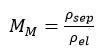(1)

Or in terms of the inverse term, namely, conductivity, it may be expressed as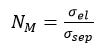(2)

This calculation is preceded by electrochemical impedance spectroscopy (EIS) to measure the resistance of the electrolyte (Rel) and of the separator (Rsep), which is used to find the resistivity by calculating their product with the geometric factors of the electrochemical cells.Download the Article

## Experimental Setup

The resistivity values are calculated based upon EIS measurements and data fitting. A Metrohm-Autolab PGSTAT204 fitted with a FRA32M module is used to perform the EIS.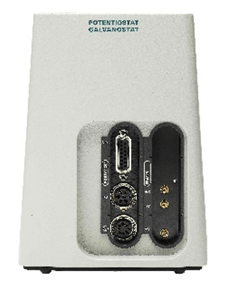Figure 1. The Metrohm-Autolab PGSTAT204, with the FRA32M module.

An Autolab RHD Microcell HC setup is also employed to regulate the experimental temperature conditions, comprising a cell holder capable of holding many different types of cells depending on the application, a Peltier element fitted into the holder, and a NOVA-dependent settable temperature controller.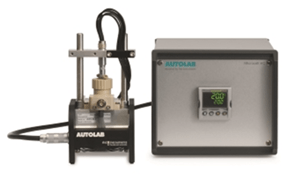Figure 2. The Autolab RHD Microcell HC setup, composed of a cell stand (left-hand side), and the temperature controller (right-hand side).

Free electrolyte resistivity is measured using a TSC1600 closed cell which has been fitted with a Pt container of 1.6 mL capacity (Figure 3A). The soaked electrolyte resistivity is measured using a TSC SW closed cell which has parallel two plane flat disk electrodes (Figure 3B). A Metrohm 3M solution of potassium chloride is used as electrolyte.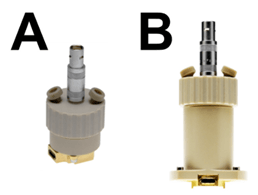Figure 3. The TSC1600 closed cell (A), and the TSC SW closed cell (B).

For free electrolyte values, the cell is filled to capacity with this solution and closed. For measuring separator resistivity, a temporary separator is made by cutting a disk of Whatman qualitative filter paper grade 1, CAS 1001090, of thickness 180 µm and pore size 11 µm, to the same diameter as the disk electrodes of the TSC SW cell and soaking it in the test electrolyte.

## The Geometric Factors

Since the geometric factors of the cells play an important role in the calculation, they must be calculated correctly. In the case of the TSC SW cell, the geometric factor Ksw is found by dividing the separator thickness by the sample area, which yields Ksw= 1.68E-4 cm-1.

The geometric factor of the TSC 1600 closed cell is more difficult to calculate, but the best way may be to first measure the resistance of a conductivity standard (Rst) with a table of conductivity values (σst), from which the geometric factor (K1600) is derived.

(K1600) = Rstst                                                                                    (3)

The EIS measurement data is then fitted to give the chosen Rst.

This experiment used the Metrohm conductivity standard of 6.2324.010, while the σst is µS cm-1 at 25 0C. The TSC 1600 cell is filled with the conductivity standard and the cell was closed and placed in the holder, to be kept at 25 0C. The two-electrode EIS readings are taken and the data are fitted with a circuit showing [RstQdl] equivalency. This gave a reading of Rst = 661 Ω. Using Equation 3, it was found that K1600 = 15.13 cm-1.

## The Procedure

The EIS measurements are taken at open circuit potential (OCP) using a two-electrode configuration after a stable temperature of 25 °C is attained, for both free electrolyte and soaked separator. At this point, frequencies begin to be applied at 10 frequencies per decade, at 10 mV amplitude, from 1 kHz to 10 Hz.

Following each measurement, the data is fitted with an [RQdl] equivalent circuit. Here R = Rel and R = Rsep, corresponding to the resistance of the free electrolyte and soaked separator respectively, and Qdl is the constant-phase element representing the double layer.

The important value is the resistance, R, which when divided by the geometric factor of each cell (K, in cm-1) yields the resistivity at the selected level, namely, ρel and ρsep respectively as Equation 4 describes. The geometric factor for the TSC 1600 and the TSC SW closed cells are represented by K1600 and Ksw respectively.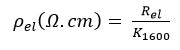(4)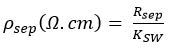## Results and Discussion

In this experiment the Nyquist plots are used to fit the data points, from which the resistance is derived. Rsep= 139 kΩ for free electrolyte (Figure 4), while Rsep = 233.35 Ω for soaked separator (Figure 5).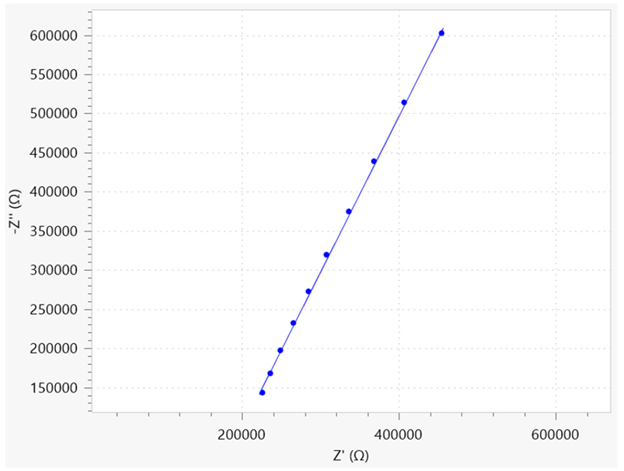Figure 4. Nyquist plot of the free electrolyte solution. The dots are referring to the data points, the solid line is the fitted result.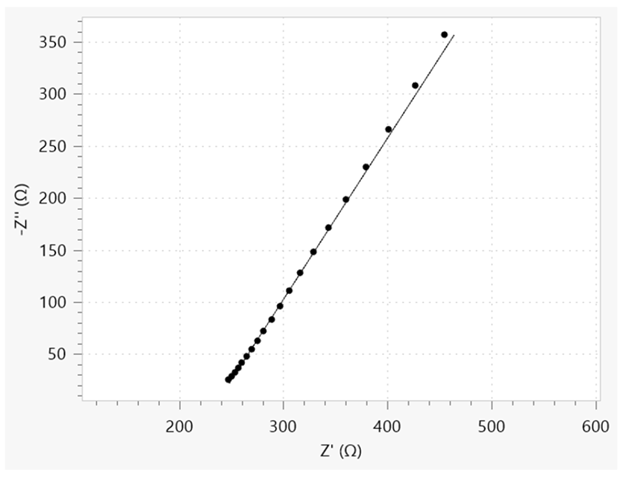Figure 5. Nyquist plot of the soaked separator. The dots are referring to the data points, while the solid line is the fitted result.

The resistivity is then calculated from Equation 4 and these values, along with cell constants and conductivity values, are listed in Table 1.

Table 1. Resistance R, resistivity ρ and conductivity σ values for the free electrolyte and the soaked separator. Also the respective cell constants K are listed.

R (Ω) K (cm-1) ρ (Ω.cm) σ (S.cm-1)
Free electrolyte (TSC 1600 closed) 1.39E5 15.13 9.92E3 1.01E-4
Soaked separator (TSC SW closed) 233.35 1.68E-4 1.39E6 7.18E-7

Using these values in Equation 1, the MacMullin number is calculated, NM = 140. This means the resistivity of the electrolyte in the porous separator is increased 140 times when compared to that of free electrolyte, or in other words, the conductivity of free electrolyte is reduced to 1/140 by the presence of the separator.

## Conclusions

The quality of an electrolyte-soaked separator in a battery cell is determined by the MacMullin number which depicts the ionic conductivity. This number is determined using data points generated from EIS measurements of two materials, fitted and multiplied by the geometric factors of the cells used for measurement.

In this experiment, a porous filter with commercial electrolyte were used to simulate battery cell conditions. The MacMullin number derived from these measurements reveals the resistivity of the electrolyte goes up much higher when it is associated with a porous separator compared to its free state.This information has been sourced, reviewed and adapted from materials provided by Metrohm AG.

## Citations

• APA

Metrohm AG. (2020, May 01). MacMullin Number Determination. AZoM. Retrieved on October 22, 2020 from https://www.azom.com/article.aspx?ArticleID=15448.

• MLA

Metrohm AG. "MacMullin Number Determination". AZoM. 22 October 2020. <https://www.azom.com/article.aspx?ArticleID=15448>.

• Chicago

Metrohm AG. "MacMullin Number Determination". AZoM. https://www.azom.com/article.aspx?ArticleID=15448. (accessed October 22, 2020).

• Harvard

Metrohm AG. 2020. MacMullin Number Determination. AZoM, viewed 22 October 2020, https://www.azom.com/article.aspx?ArticleID=15448.

## Tell Us What You Think